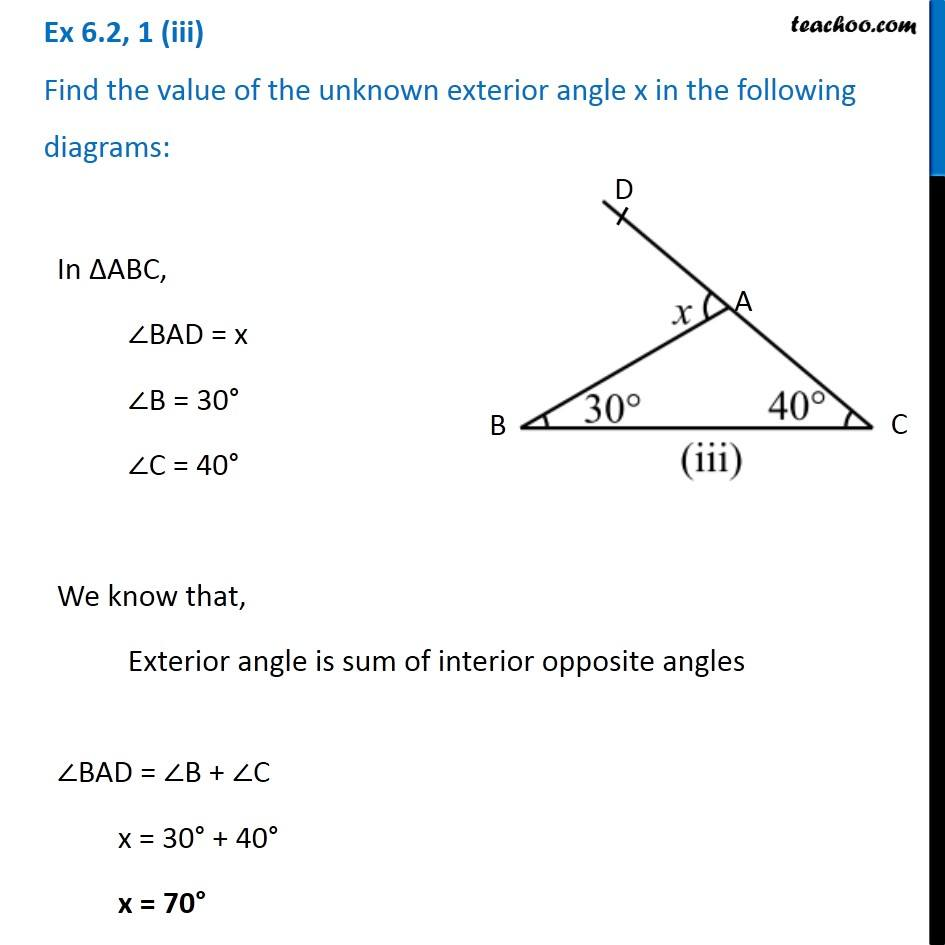1. Chapter 6 Class 7 Triangle and its Properties
2. Serial order wise
3. Ex 6.2

Transcript

Ex 6.2, 1 (iii) Find the value of the unknown exterior angle x in the following diagrams: In ∆ABC, ∠BAD = x ∠B = 30° ∠C = 40° We know that, Exterior angle is sum of interior opposite angles ∠BAD = ∠B + ∠C x = 30° + 40° x = 70°